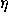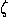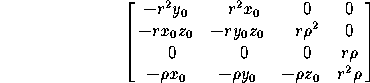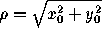# 11.3 Projective Transformations

A transformation that maps lines to lines (but does not necessarily preserve parallelism) is a projective transformation. Any spatial projective transformation can be expressed by an invertible 4×4 matrix in homogeneous coordinates; conversely, any invertible 4×4 matrix defines a projective transformation of the plane. Projective transformations (if not affine) are not defined on all of space, but only on the complement of a plane (the missing plane is ``mapped to infinity'').

The following particular case is often useful, especially in computer graphics, in projecting a scene from space to the plane. Suppose an observer is at the point E=(x,y,z) of space, looking toward the origin O=(0,0,0). Let P, the screen, be the plane through O and perpendicular to the ray EO. Place a rectangular coordinate systemon P with origin at O and such that the positive-axis lies in the half-plane determined by E and the positive z-axis of space (that is, the z-axis is pointing ``up'' as seen from E). Then consider the transformation that associates to a point X=(x,y,z) the triple (,,), where (,) are the coordinates of the point where the line EX intersects P (the screen coordinates of X as seen from E), andis the inverse of the signed distance from X to E along the line EO (the depth of X as seen from E). This is a projective transformation, given by the matrixwithand.

Next: 12 DirectionsPlanes and Lines
Up: 11 Other Transformations of Space
Previous: 11.2 Affine TransformationsThe Geometry Center Home Page

Silvio Levy
Wed Oct 4 16:41:25 PDT 1995

This document is excerpted from the 30th Edition of the CRC Standard Mathematical Tables and Formulas (CRC Press). Unauthorized duplication is forbidden.